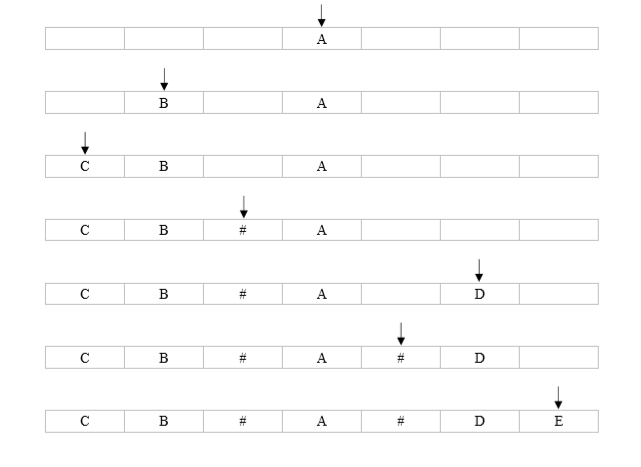# Case1:满二叉树

## 示例1

```Sample Input:

## Solution：

根节点插入到数组中间位置，

左子树根节点插入到数组左边的中间位置，

一直这样直至数组左边中间位置已满开始插入到右边数组的中间位置，

依次递归 . . . . . .```#include <iostream>
#include <string.h>

using namespace std;

int i;                                              // 全局变量，控制字符串的遍历
string s;

void insert(char a[], int left, int right){         // 将字符串各个元素插入数组对应位置
int mid = (left + right) / 2;
if(left <= right && a[mid] != s[i]){
a[mid] = s[i++];
insert(a, left, mid-1);
insert(a, mid+1, right);
}
}

bool cimi2(int x){                                  // 判断x是否是2的n次幂
return (x&(x-1))?false:true;
}

int main(){
while(cin>>s){
int n = s.length();
if(!cimi2(n+1)){                            // 判断是否是满二叉树
cout<<"不满足满二叉树"<<endl;
}
else{
char *A = new char[n];                  // 动态分配字符串数组
i = 0;
int left = 0, right = n-1;
insert(A, left, right);
for(int j = 0; j < strlen(A); j++){
if(A[j] != '#'){
cout<<A[j]<<" ";
}
}
cout<<endl;
delete [] A;                            // 回收动态分配的字符串数组
}
}
return 0;
}```

# Case2:无限制条件的二叉树

## 示例1

`abc##de#g##f###`

`c b e g d f a `

## Solution1:

```#include <iostream>
#include <string>
using namespace std;
string str;
int i;
struct TreeNode
{
char val;
struct TreeNode *lchild, *rchild;
TreeNode(char c) :val(c), lchild(NULL), rchild(NULL) {}
};
TreeNode* createTree() {
char c = str[i++];
if (c == '#') return NULL;
TreeNode *root = new TreeNode(c);     // 动态分配一个TreeNode类型存储空间，返回类型为指针
root->lchild = createTree();
root->rchild = createTree();
return root;                          // 返回动态分配的指针
}
void inOrderTraversal(TreeNode* root) {
if (!root) return;
inOrderTraversal(root->lchild);
cout << root->val << " ";
inOrderTraversal(root->rchild);
}
int main() {
while (cin >> str) {
i = 0;
TreeNode *root = createTree();
inOrderTraversal(root);
cout << endl;　　　　　delete [] root;     // 动态分配的存储空间要回收
}
return 0;
}```

## Solution2:

```//这个实际上就是树的遍历的非递归实现，入栈时访问 = 前序， 出栈时访问 = 中序
#include <iostream>
#include <string>
#include <stack>
using namespace std;
int main()
{  string pre;  while(cin >> pre){
stack<char> s;
for(auto it : pre){
if(it != '#')
s.push(it);
else{
if(!s.empty()){
cout << s.top() << ' ';
s.pop();
}
}
}
cout << '\n';  }
}```